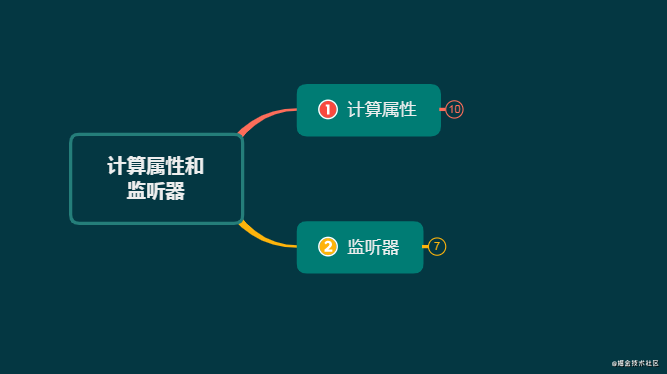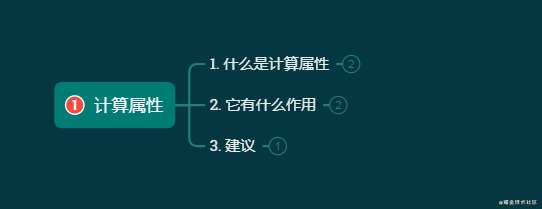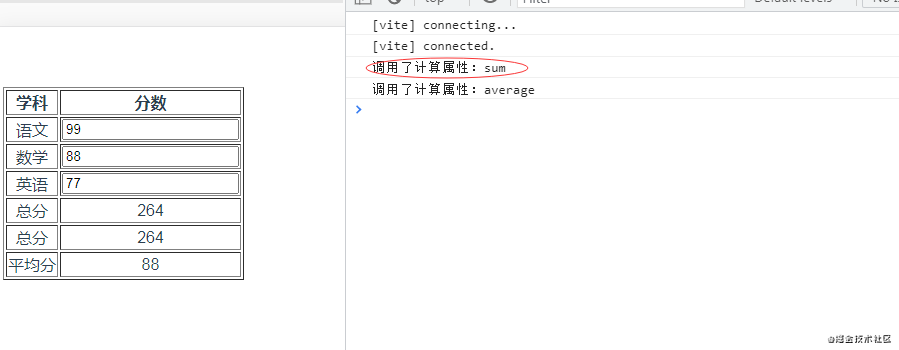# 理解计算属性和监听器 | 重学Vue3image.png

# 计算属性image.png

1. 什么是计算属性

``````{
//...
computed:{}
}
``````

``````  computed: {
//方法
sum(){
//...
},
//对象
average: {
// getter
get() {
//...
},
// setter
set(newValue) {
//...
}
}
}
``````

2. 它有什么作用

``````

学科
分数

语文

数学

英语

总分
{{math + English + Chinese}}

平均分
{{(math + English + Chinese)/3}}

export default {
data() {
return {
math: 88,
English: 77,
Chinese: 99,
}
}
}

``````

``````computed: {
sum() {
console.log('调用了计算属性：sum');
return this.math + this.English + this.Chinese;
},
average() {
console.log('调用了计算属性：average');
return Math.round(this.sum / 3);
}
}
``````

``````
总分
{{sum}}

平均分
{{average}}

``````

``````
总分
{{sum}}

总分
{{sum}}

``````image.png

3. 建议

# 监听器

[图片上传失败…(image-a891e1-1627130855151)]

1. 什么是监听器

``````{
data() {
return {
count:1
}
},
watch: {
//...
}
}
``````

``````watch: {
count(){...},
//...
}
``````

2. 它有什么作用

``````
{{ count }}

export default {
name: "HelloWorld",
data() {
return {
count: 0
}
},
watch:{
count(val){
console.log('执行了监听器count');
}
},
mounted(){
this.count ++
}
}

``````

``````

``````
``````

const watchExampleVM = Vue.createApp({
data() {
return {
question: '',
answer: 'Questions usually contain a question mark. ;-)'
}
},
watch: {
// whenever question changes, this function will run
question(newQuestion, oldQuestion) {
if (newQuestion.indexOf('?') > -1) {
}
}
},
methods: {
axios
.get('https://yesno.wtf/api')
.then(response => {
})
.catch(error => {
this.answer = 'Error! Could not reach the API. ' + error
})
}
}
}).mount('#watch-example')

``````

3. `\$watch`

`Vue`在组件实例上内置了`\$watch`属性，功能其实与配置选项`watch`一样，都是用于监听属性

`END~`